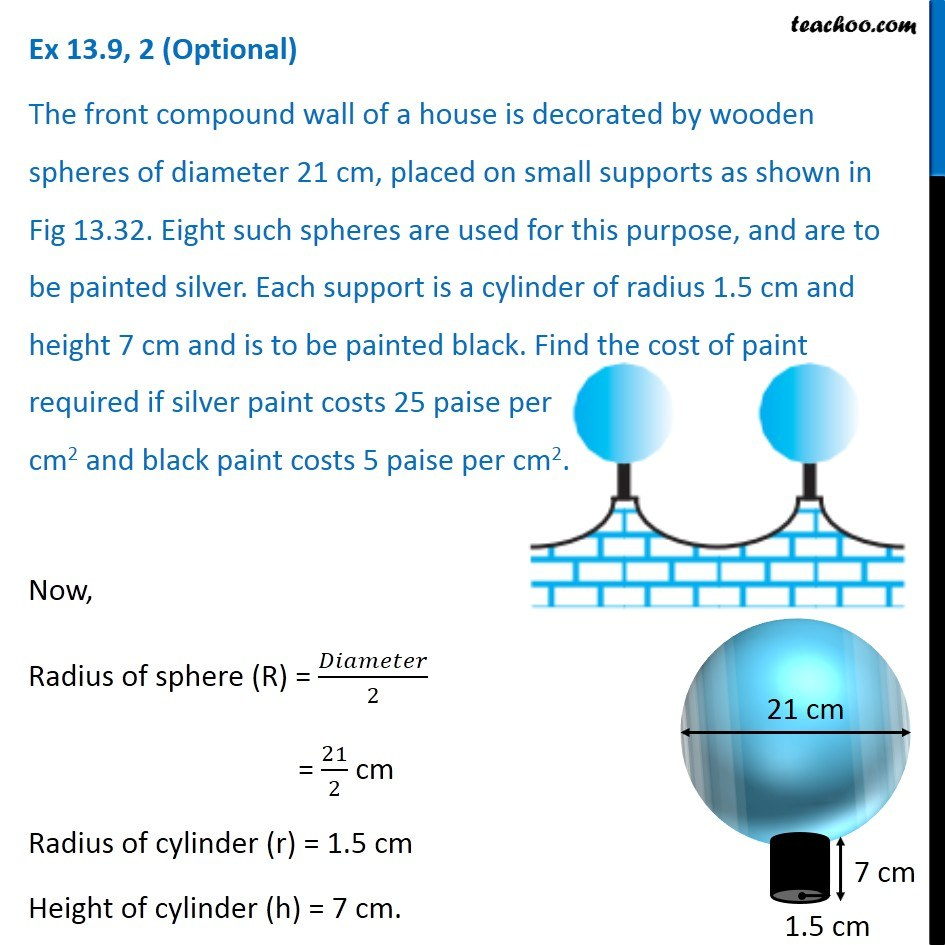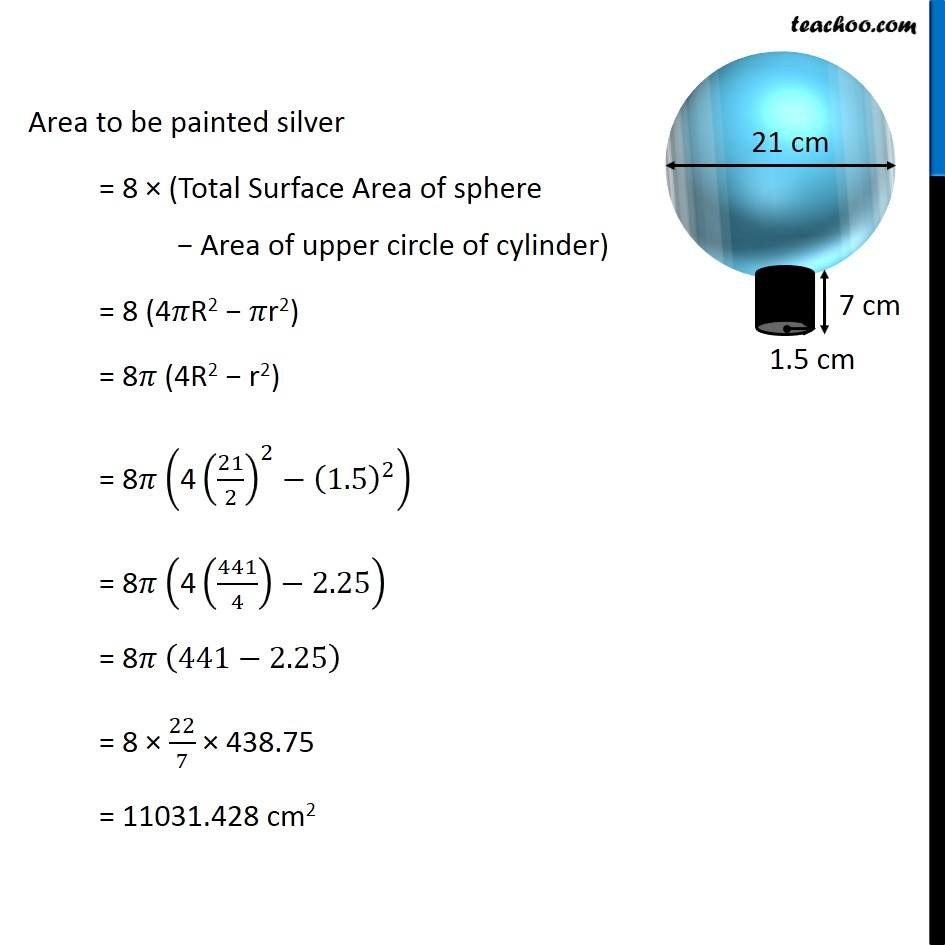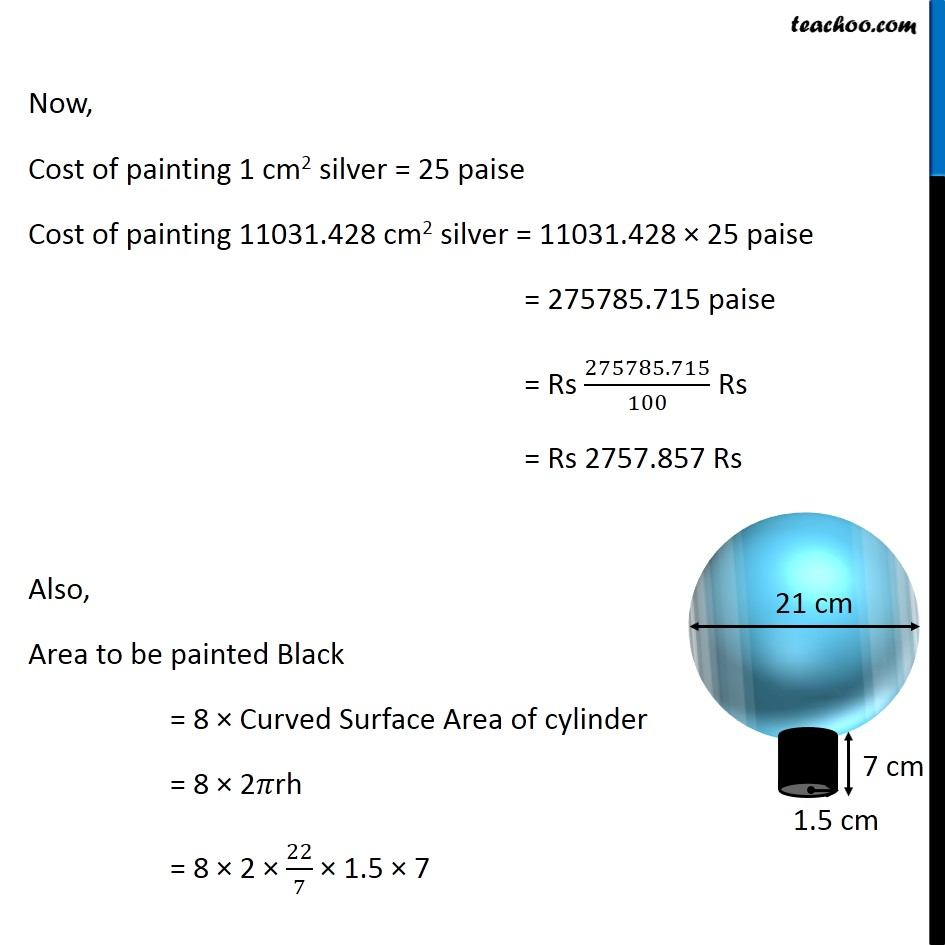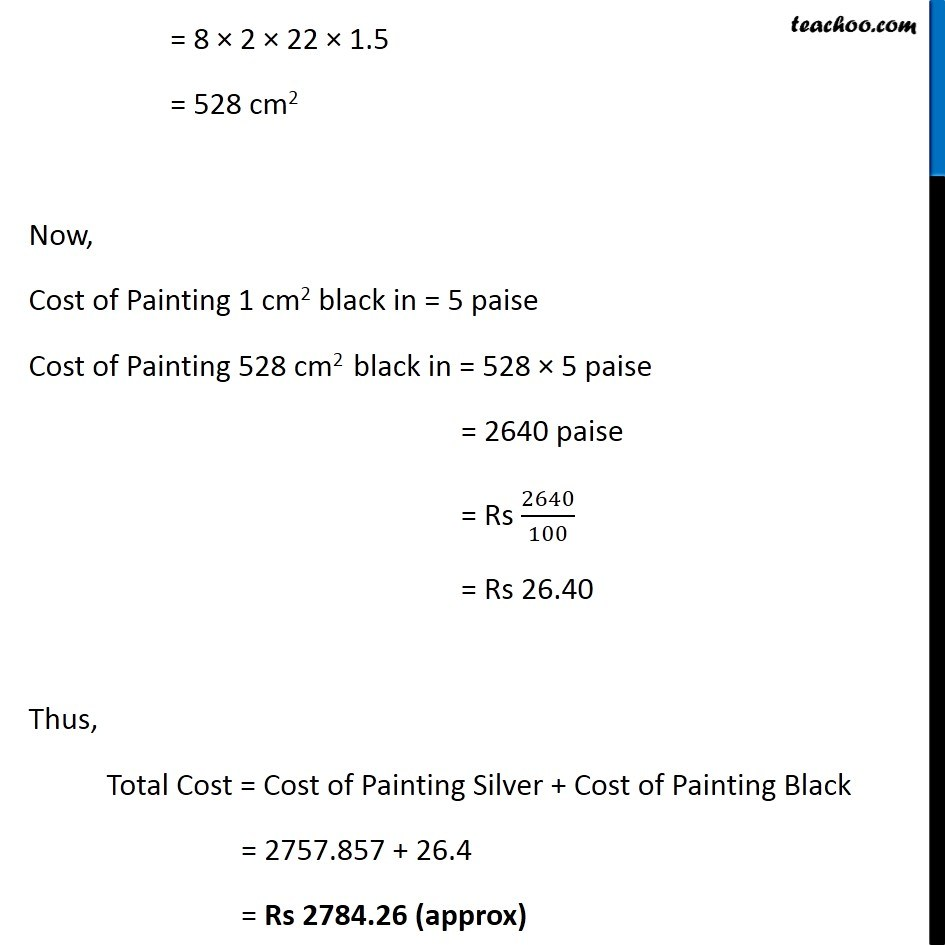Important Surface Area QuestionsLearn in your speed, with individual attention - Teachoo Maths 1-on-1 Class

### Transcript

Question 2 The front compound wall of a house is decorated by wooden spheres of diameter 21 cm, placed on small supports as shown in Fig 13.32. Eight such spheres are used for this purpose, and are to be painted silver. Each support is a cylinder of radius 1.5 cm and height 7 cm and is to be painted black. Find the cost of paint required if silver paint costs 25 paise per cm2 and black paint costs 5 paise per cm2. Now, Radius of sphere (R) = 𝐷𝑖𝑎𝑚𝑒𝑡𝑒𝑟/2 = 21/2 cm Radius of cylinder (r) = 1.5 cm Height of cylinder (h) = 7 cm. Area to be painted silver = 8 × (Total Surface Area of sphere − Area of upper circle of cylinder) = 8 (4𝜋R2 − 𝜋r2) = 8𝜋 (4R2 − r2) = 8𝜋 ("4" (21/2)^2−(1.5)^2 ) = 8𝜋 ("4" (441/4)−2.25) = 8𝜋 ("441" −2.25) = 8 × 22/7 × 438.75 = 11031.428 cm2 Now, Cost of painting 1 cm2 silver = 25 paise Cost of painting 11031.428 cm2 silver = 11031.428 × 25 paise = 275785.715 paise = Rs 275785.715/100 Rs = Rs 2757.857 Rs Also, Area to be painted Black = 8 × Curved Surface Area of cylinder = 8 × 2𝜋rh = 8 × 2 × 22/7 × 1.5 × 7 = 8 × 2 × 22 × 1.5 = 528 cm2 Now, Cost of Painting 1 cm2 black in = 5 paise Cost of Painting 528 cm2 black in = 528 × 5 paise = 2640 paise = Rs 2640/100 = Rs 26.40 Thus, Total Cost = Cost of Painting Silver + Cost of Painting Black = 2757.857 + 26.4 = Rs 2784.26 (approx)